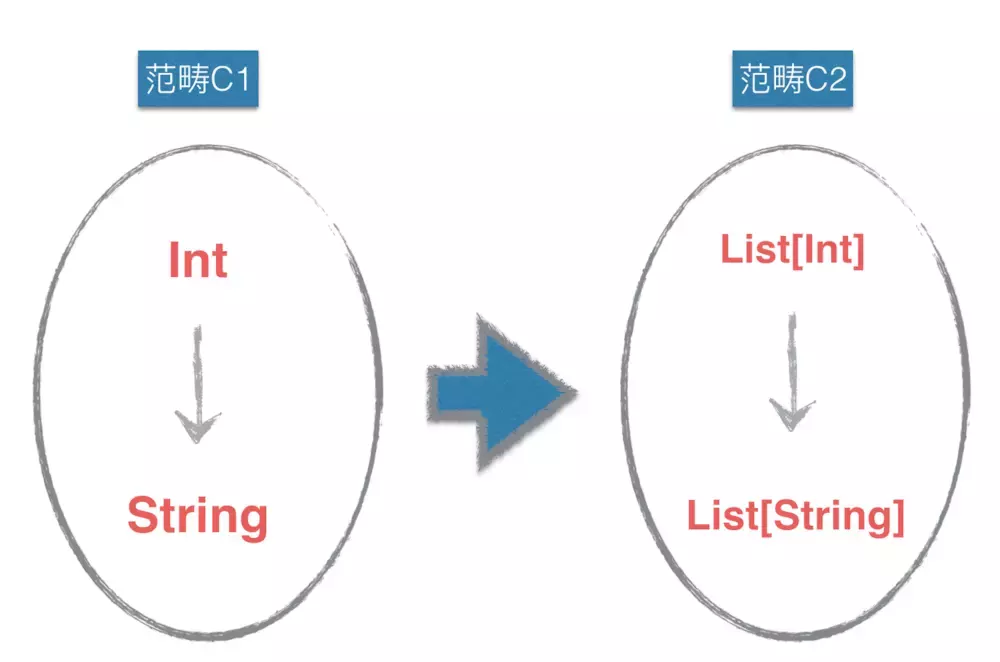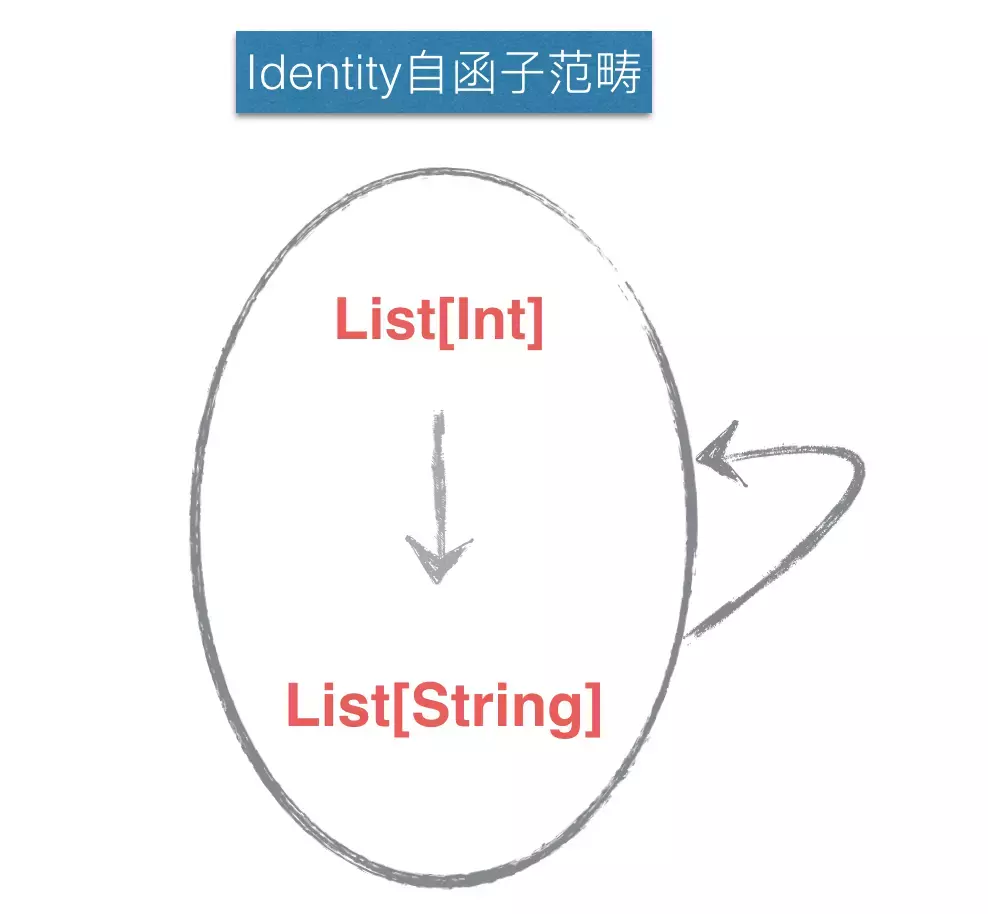# 自函数 EndoFunction

``````identity :: Number -> Number
``````

identity函数就是一个自函数的例子，它接收什么就返回什么

# 函子如何映射两个范畴Haskell中，Functor是可以被map over的东西，List就是一个典型的instance。构造List[Int] 就是把Int提升到List[Int]，记作：`Int -> List[Int]` . 这表达了一个范畴的元素可以被映射为另一个范畴的元素

``````map :: (a -> b) -> [a] -> [b]
``````

``````map :: (Int -> String) -> (List[Int] -> List[String])
``````

map的定义清晰的告诉我们： `Int -> String` 这个关系可以被映射为 `List[Int] -> List[String]` 这种关系。这就表达了元素间的关系可以映射为另外一个范畴元素间的关系

# 自函子``````类型List[Int] 映射到自己

``````

``````F(List[Int]) = List[Int]
F(f) = f

``````

# 幺半群

``````(1) 封闭性：（Closure）：对于任意a，b∈G，有a*b∈G
(2) 结合律（Associativity）：对于任意a，b，c∈G，有（a*b）*c=a*（b*c）
(3) 幺元 （Identity）：存在幺元e，使得对于任意a∈G，e*a=a*e=a
(4) 逆元：对于任意a∈G，存在逆元a^-1，使得a^-1*a=a*a^-1=e
``````

``````cube :: Number -> Number
``````

``````f :: Number -> (Number, String)
``````

``````(.) :: (b -> c) -> (a -> b) -> a -> c

f . f
``````

``````F Number = (Number, String)
F Number -> Number = (Number,String) -> (Number,String)
``````

``````fn :: (Number,String) -> (Number,String)
fn . fn
``````

``````liftM :: (Double -> (Double, String)) -> (Double,String) -> (Double, String)
liftM f (x,y) = case r of (n,s) -> (n, y ++ s)
where r = f x
``````

``````cube :: Number -> (Number, String)
cube x = (x * x * x, "cube was called.")

sine :: Number -> (Number, String)
sine x = (Math.sin x, "sine was called.")

f = ((liftM sine) . (liftM cube)) . (liftM cube)
f (3, "")

f1 = (liftM sine) . ((liftM cube) . (liftM cube))

``````

``````unit :: Number -> (Number, String)
unit x = (x, "")

f = (liftM sine) . (liftM cube)

f . (liftM unit) = (liftM unit) . f = f
``````

`unit` 个人理解应该就是类型构造器